###### back to index | new

Suppose that the angles of $\triangle ABC$ satisfy $cos(3A)+cos(3B)+cos(3C)=1.$ Two sides of the triangle have lengths $10$ and $13$. There is a positive integer $m$ so that the maximum possible length for the remaining side of $\triangle ABC$ is $\sqrt{m}$. Find $m$.

Let $A,B,C$ be angles of an acute triangle with $$\cos^2 A + \cos^2 B + 2 \sin A \sin B \cos C = \frac{15}{8}$$ and $$\cos^2 B + \cos^2 C + 2 \sin B \sin C \cos A = \frac{14}{9}$$ There are positive integers $p$, $q$, $r$, and $s$ for which $\cos^2 C + \cos^2 A + 2 \sin C \sin A \cos B = \frac{p-q\sqrt{r}}{s},$ where $p+q$ and $s$ are relatively prime and $r$ is not divisible by the square of any prime. Find $p+q+r+s$.

The angles in a particular triangle are in arithmetic progression, and the side lengths are $4,5,x$. The sum of the possible values of x equals $a+\sqrt{b}+\sqrt{c}$ where $a, b$, and $c$ are positive integers. What is $a+b+c$?

In the figure shown below, $ABCDE$ is a regular pentagon and $AG=1$. What is $FG + JH + CD$?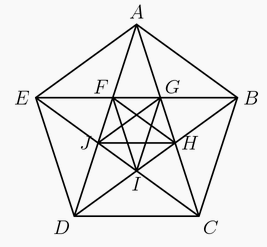An infinite number of equilateral triangles are constructed as shown on the right. Each inner triangle is inscribed in its immediate outsider and is shifted by a constant angle $\beta$. If the area of the biggest triangle equals to the sum of areas of all the other triangles, find the value of $\beta$ in terms of degrees.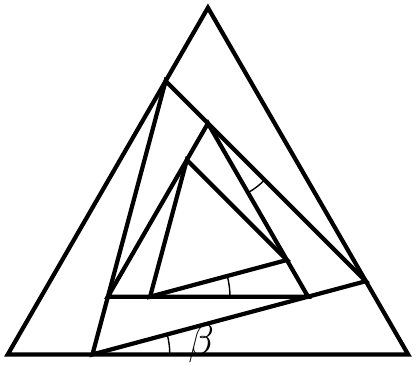As shown, prove $$\frac{\sin(\alpha+\beta)}{PC}=\frac{\sin{\alpha}}{PB}+\frac{\sin{\beta}}{PA}$$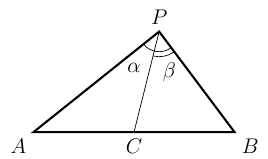In $\triangle{ABC}$, $\angle{BAC} = 40^\circ$ and $\angle{ABC} = 60^\circ$. Points $D$ and $E$ are on sides $AC$ and $AB$, respectively, such that $\angle{DBC}=40^\circ$ and $\angle{ECB}=70^\circ$. Let $F$ be the intersection point of $BD$ and $CE$. Show that $AF\perp BC$.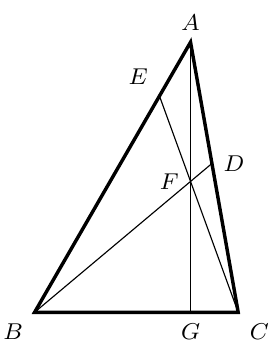In $\triangle{ABC}$ show that $$\tan nA + \tan nB + \tan nC = \tan nA \tan nB \tan nC$$ where $n$ is an integer.

In $\triangle{ABC}$, if $A:B:C=4:2:1$, prove $$\frac{1}{a}+\frac{1}{b}=\frac{1}{c}$$

In acute $\triangle{ABC}$, $\angle{ACB}=2\angle{ABC}$. Let $D$ be a point on $BC$ such that $\angle{ABC}=2\angle{BAD}$. Show that $$\frac{1}{BD}=\frac{1}{AB}+\frac{1}{AC}$$

As shown.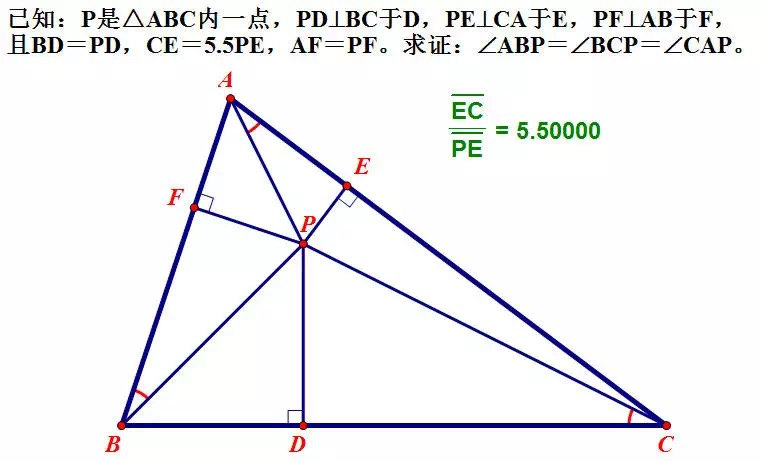Let $O$ be a point inside a convex pentagon, as shown, such that $\angle{1} = \angle{2}, \angle{3} = \angle{4}, \angle{5} = \angle{6},$ and $\angle{7} = \angle{8}$. Show that either $\angle{9} = \angle{10}$ or $\angle{9} + \angle{10} = 180^\circ$ holds.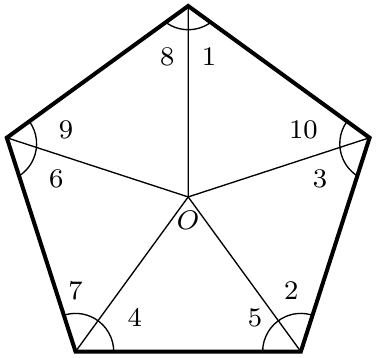In $\triangle{ABC}$, $AE$ and $AF$ trisects $\angle{A}$, $BF$ and $BD$ trisects $\angle{B}$, $CD$ and $CE$ trisects $\angle{C}$. Show that $\triangle{DEF}$ is equilateral.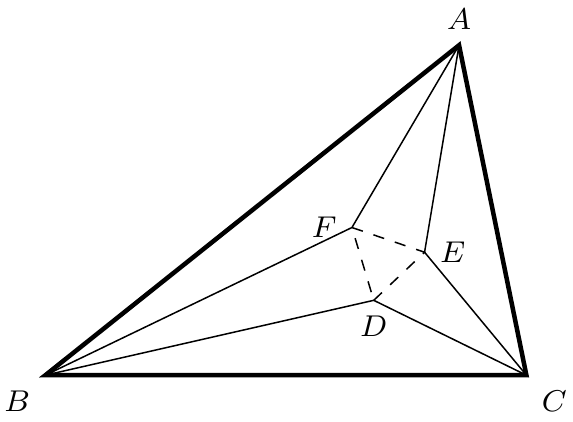As shown, $\angle{ACB} = 90^\circ$, $AD=DB$, $DE=DC$, $EM\perp AB$, and $EN\perp CD$. Prove $$MN\cdot AB = AC\cdot CB$$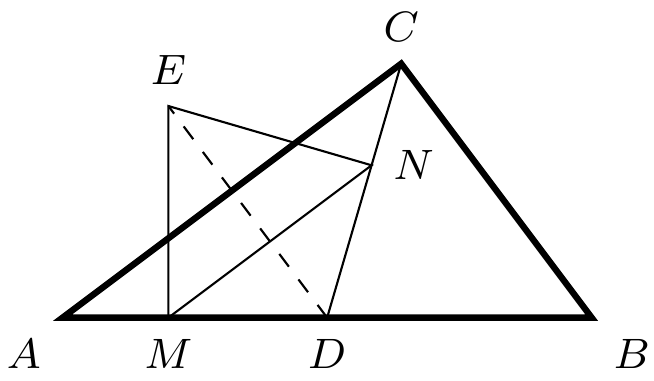As shown, in $\triangle{ABC}$, $AB=AC$, $\angle{A} = 20^\circ$, $\angle{ABE} = 30^\circ$, and $\angle{ACD}=20^\circ$. Find the measurement of $\angle{CDE}$.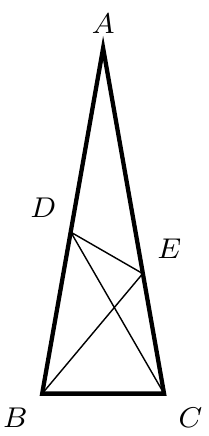Let $A, B,$ and $C$ be angles of a triangle. If $\cos 3A + \cos 3B + \cos 3C = 1$, determine the largest angle of the triangle.

In $\triangle{ABC}$, show that $$\sin\frac{A}{2}=\sqrt{\frac{(p-b)(p-c)}{bc}}$$ where $p=\frac{a+b+c}{2}$ is the semi-perimeter.

In $\triangle{ABC}$, show that \begin{align*} \sin 2A + \sin 2B + \sin 2C &= 4\sin A\sin B \sin C\\ \cos 2A + \cos 2B + \cos 2C &= -1-4\cos A\cos B\cos C \end{align*}

In $\triangle{ABC}$, show that \begin{align*} &\sin^2A +\sin^2B+\sin^2C = 2 +2\cos A\cos B \cos C\\ &\cos^2A +\cos^2B + \cos^2C = 1-2\cos A\cos B\cos C \end{align*}